### Day 08 - Limits Problems - 08.27.14

 Bell RingerMissing Exit Tickets?Create a problem involving one of the concepts covered in chapter 1. Include an answer key with multiple ways to solve the problem.Evaluating Limits AlgebraicallyEvaluating Limits GraphicallyEvaluating Limits NumericallyRemovable DiscontinuityNonremovable DiscontinuityOne-sided LimitsInfinite LimitsStump Your Teacher ProblemsReviewFinding limits using a tableFinding limits using a graphEpsilon-Delta Limit ProofsLimit PropertiesDividing Out/Rationalizing TechniquesFunctions that agree at all but one pointOne-sided LimitsInfinite LimitsLessonFind the values of the constants a and b such that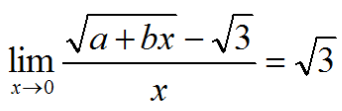Consider the function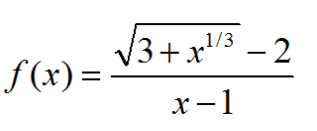Find the domain of f.Calculate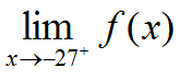Calculate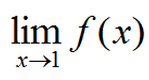Determine all value of the constant a such that the following function is continuous for all real numbers.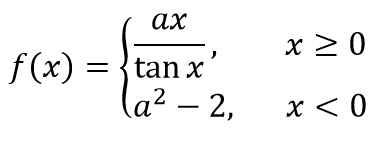Exit TicketThe function f and its graph are shown below:Calculate the limit of f(x) as x gets closer to 2 from the left side.Which value is greater?the limit of f(x) as x goes to 1f(1)Explain your answer.At what value(s) of c on the interval [0, 4] does the limit of f(x) as x goes to c not exist?Explain your answer.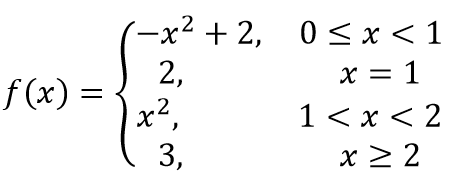Lesson Objective(s) How are the concepts in chapter 1 related? Standard(s) APC.2 Define and apply the properties of limits of functions. Limits will be evaluated graphically and algebraically. Includes: ​limits of a constant ​limits of a sum, product, and quotient ​one-sided limits ​limits at infinity, infinite limits, and non-existent limits*APC.3Use limits to define continuity and determine where a function is continuous or discontinuous.Includes:​continuity in terms of limitscontinuity at a point and over a closed interval​application of the Intermediate Value Theorem and the Extreme Value Theorem​geometric understanding and interpretation of continuity and discontinuityAPC.4Investigate asymptotic and unbounded behavior in functions.Includes:describing and understanding asymptotes in terms of graphical behavior and limits involving infinity